Date: Thu, 21 Jan 1999 17:00:26 -0600 Hi my name is Eric and I m in 8th Grade honors math (middle) and I'd like to know how to prove the quadratic formula -b±sqrt of b(a)(c)over 2(a) Hi Eric, The standard way to derive the general quadratic formula is to "complete the square". Here is an example. Solve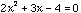First divide by the coefficient of x squared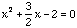To complete the square add, to both sides, the square of half the coefficient of x.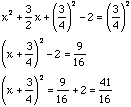Thus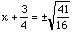, or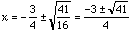To derive the formula consider the equation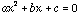and go through the same steps as in the example Cheers Penny Go to Math Central To return to the previous page use your browser's back button.